Transpose of a matrix

Chapter 3 Class 12 Matrices (Term 1)
Concept wise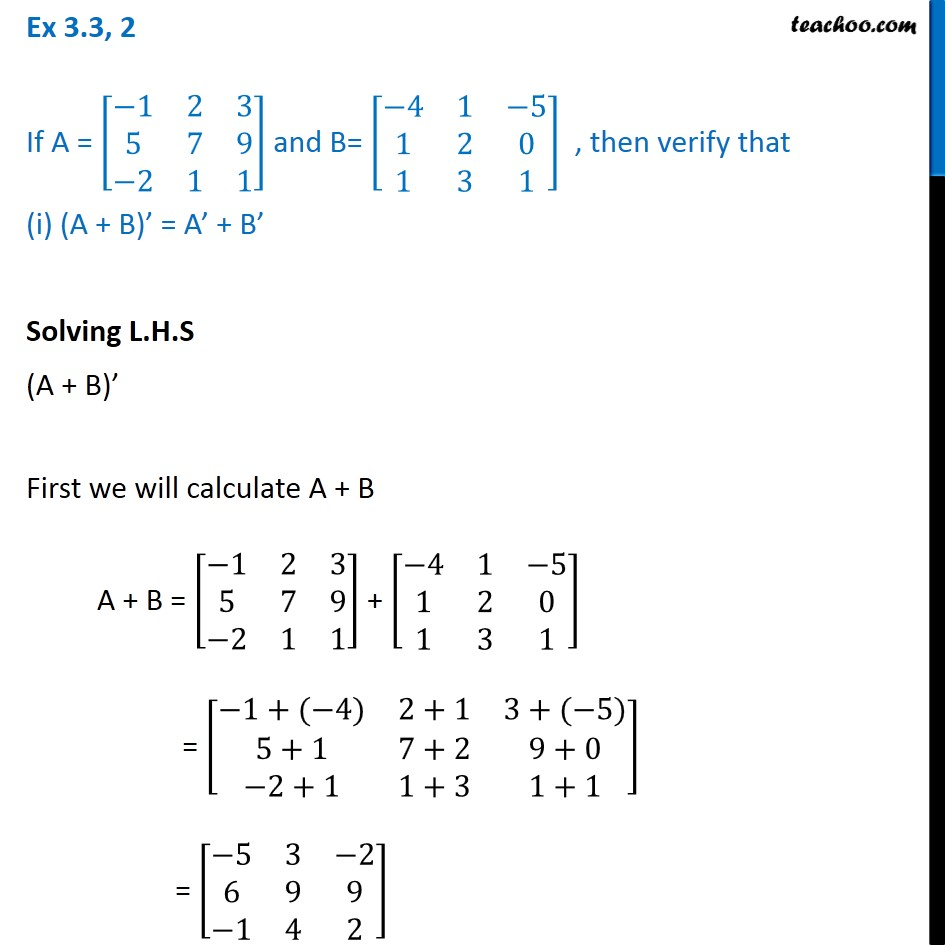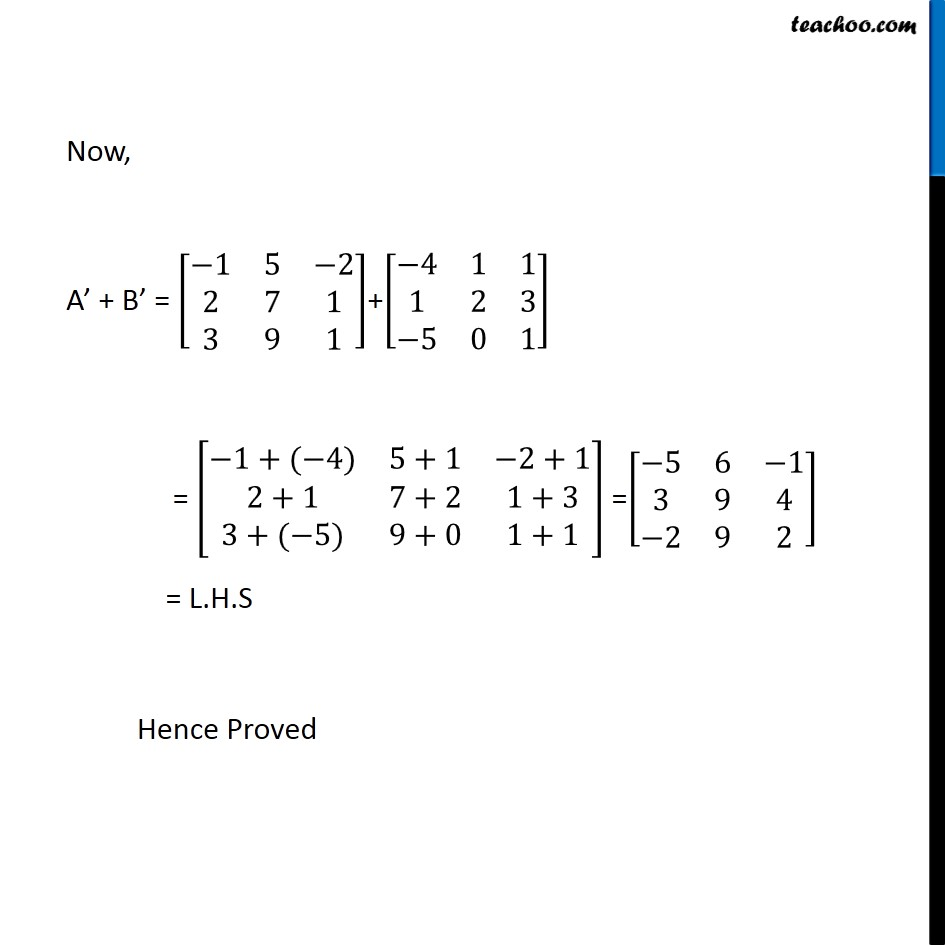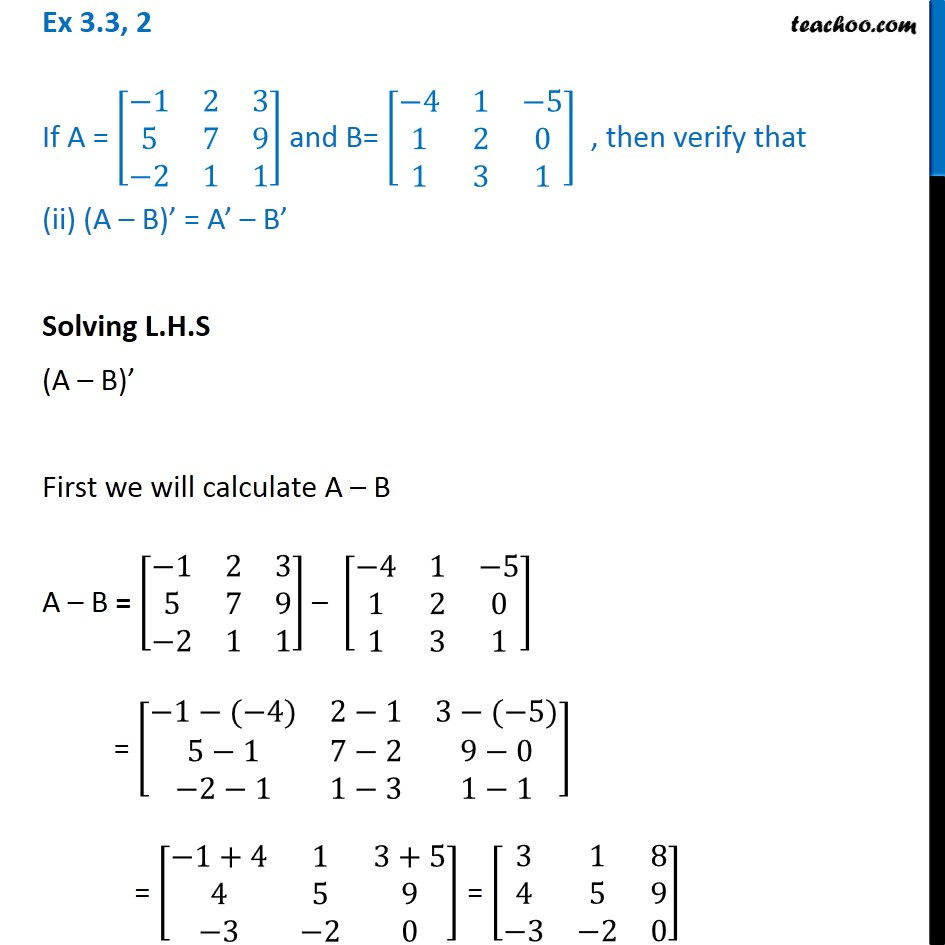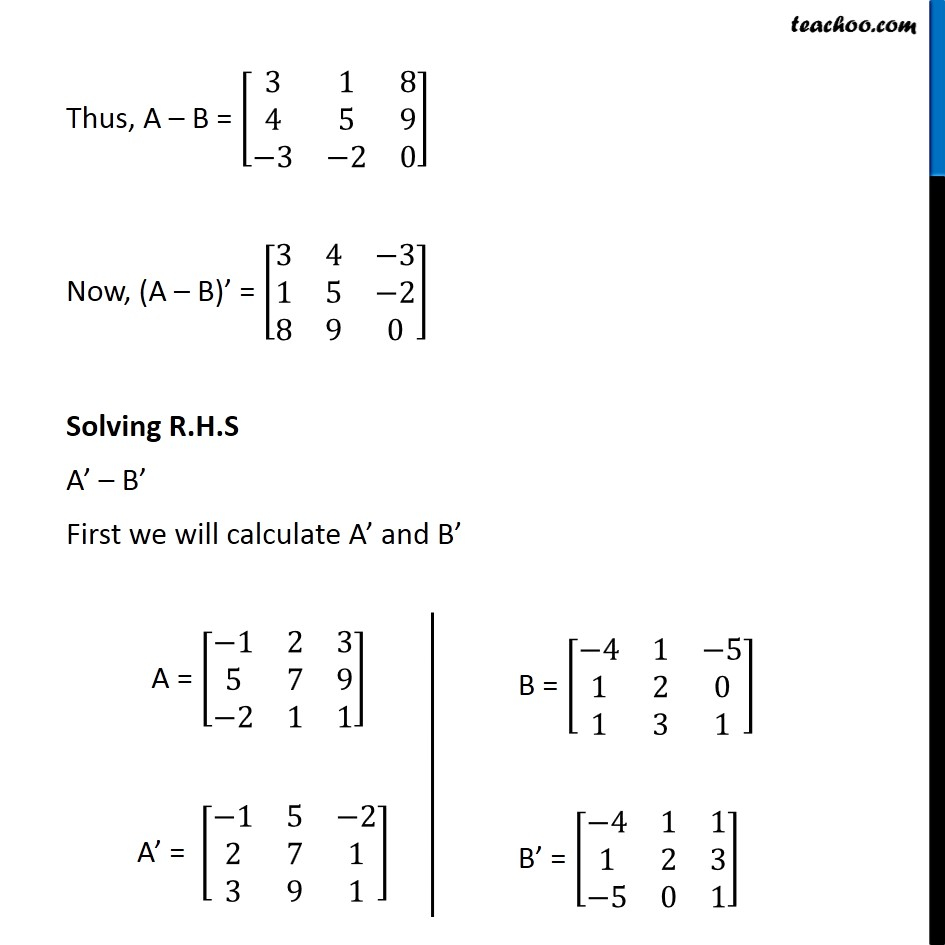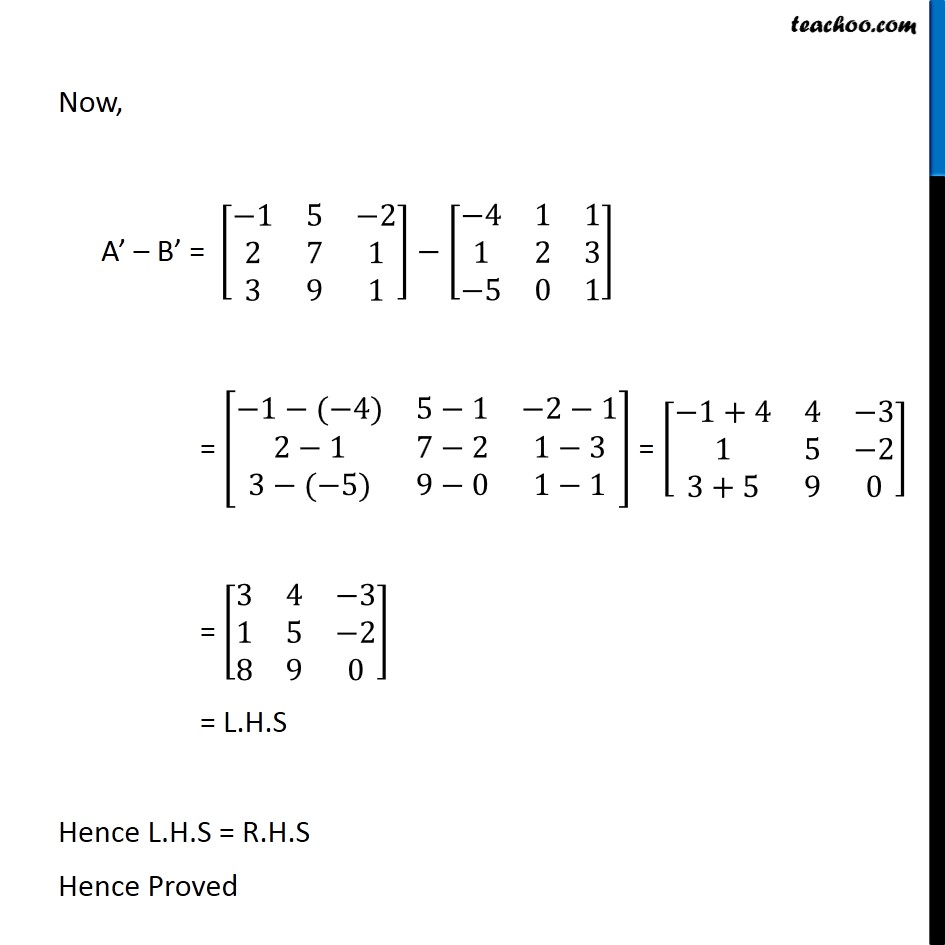### Transcript

Ex 3.3, 2 If A = [■8(−1&2&3@5&7&9@−2&1&1)] and B= [■8(−4&1&−5@1&2&0@1&3&1)] , then verify that (i) (A + B)’ = A’ + B’ Solving L.H.S (A + B)’ First we will calculate A + B A + B = [■8(−1&2&3@5&7&9@−2&1&1)] + [■8(−4&1&−5@1&2&0@1&3&1)] = [■8(−1+(−4)&2+1&3+(−5)@5+1&7+2&9+0@−2+1&1+3&1+1)] = [■8(−5&3&−2@6&9&9@−1&4&2)] Thus, A + B = [■8(−5&3&−2@6&9&9@−1&4&2)] (A + B)’ = [■8(−5&6&−1@3&9&4@−2&9&2)] Solving R.H.S A’ + B’ First we will calculate A’ and B’ A = [■8(−1&2&3@5&7&9@−2&1&1)] A’ =[■8(−1&5&−2@2&7&1@3&9&1)] B = [■8(−4&1&−5@1&2&0@1&3&1)] B’ = [■8(−4&1&1@1&2&3@−5&0&1)] Now, A’ + B’ = [■8(−1&5&−2@2&7&1@3&9&1)]+[■8(−4&1&1@1&2&3@−5&0&1)] = [■8(−1+(−4)&5+1&−2+1@2+1&7+2&1+3@3+(−5)&9+0&1+1)] =[■8(−5&6&−1@3&9&4@−2&9&2)] = L.H.S Hence Proved Ex 3.3, 2 If A = [■8(−1&2&3@5&7&9@−2&1&1)] and B= [■8(−4&1&−5@1&2&0@1&3&1)] , then verify that (ii) (A – B)’ = A’ – B’ Solving L.H.S (A – B)’ First we will calculate A – B A – B = [■8(−1&2&3@5&7&9@−2&1&1)] – [■8(−4&1&−5@1&2&0@1&3&1)] = [■8(−1−(−4)&2−1&3−(−5)@5−1&7−2&9−0@−2−1&1−3&1−1)] = [■8(−1+4&1&3+5@4&5&9@−3&−2&0)] = [■8(3&1&8@4&5&9@−3&−2&0)] Thus, A – B = [■8(3&1&8@4&5&9@−3&−2&0)] Now, (A – B)’ = [■8(3&4&−3@1&5&−2@8&9&0)] Solving R.H.S A’ – B’ First we will calculate A’ and B’ A = [■8(−1&2&3@5&7&9@−2&1&1)] A’ = [■8(−1&5&−2@2&7&1@3&9&1)] B = [■8(−4&1&−5@1&2&0@1&3&1)] B’ = [■8(−4&1&1@1&2&3@−5&0&1)] Now, A’ – B’ = [■8(−1&5&−2@2&7&1@3&9&1)]−[■8(−4&1&1@1&2&3@−5&0&1)] = [■8(−1−(−4)&5−1&−2−1@2−1&7−2&1−3@3−(−5)&9−0&1−1)] = [■8(−1+4&4&−3@1&5&−2@3+5&9&0)] = [■8(3&4&−3@1&5&−2@8&9&0)] = L.H.S Hence L.H.S = R.H.S Hence Proved